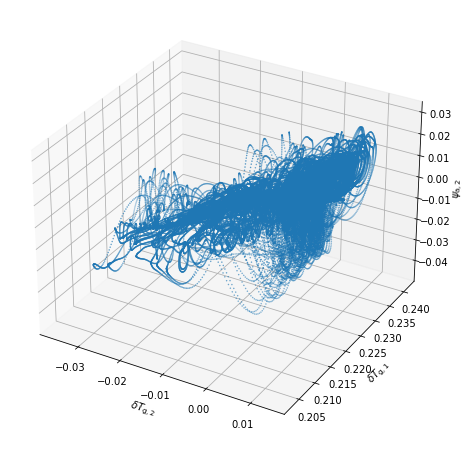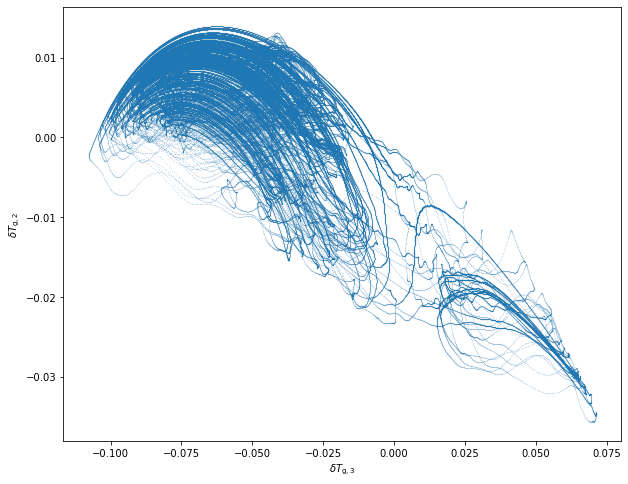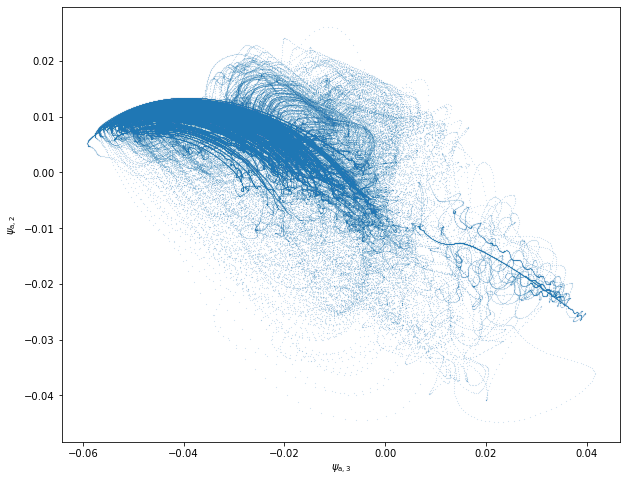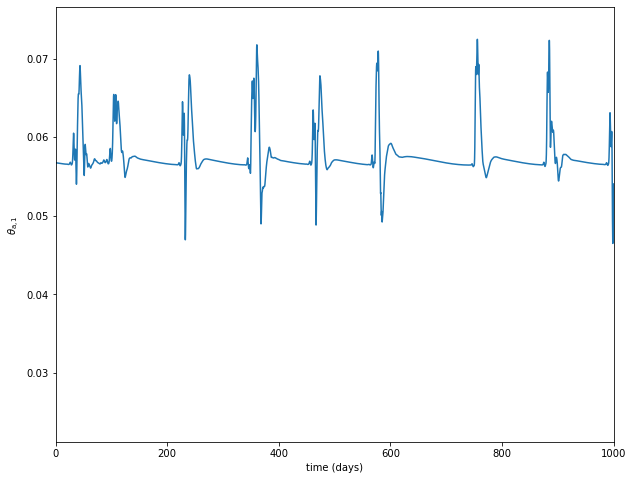# Atmospheric model with heat exchange - Li et al. model version (2017)

This model version is a simple 2-layer channel QG atmosphere truncated at wavenumber 2 on a beta-plane with a simple orography (a montain and a valley) , with a radiative and heat exchanges scheme inspired by the MAOOAM model.

More detail can be found in the articles:

• Li, D., He, Y., Huang, J., Bi, L., & Ding, L. (2018). Multiple equilibria in a land–atmosphere coupled system. Journal of Meteorological Research, 32(6), 950-973. doi:10.1007/s13351-018-8012-y

• De Cruz, L., Demaeyer, J. and Vannitsem, S. (2016). The Modular Arbitrary-Order Ocean-Atmosphere Model: MAOOAM v1.0, Geosci. Model Dev., 9, 2793-2808. doi:10.5194/gmd-9-2793-2016

## Modules import

import numpy as np
import matplotlib.pyplot as plt
from mpl_toolkits.mplot3d import Axes3D


Initializing the random number generator (for reproducibility). – Disable if needed.

np.random.seed(210217)


Importing the model’s modules

from qgs.params.params import QgParams
from qgs.integrators.integrator import RungeKuttaIntegrator, RungeKuttaTglsIntegrator
from qgs.functions.tendencies import create_tendencies
from qgs.plotting.util import std_plot


## Systems definition

General parameters

# Time parameters
dt = 0.1
# Saving the model state n steps
write_steps = 5

number_of_trajectories = 1
number_of_perturbed_trajectories = 10


Setting some model parameters

# Model parameters instantiation with some non-default specs
# Mode truncation at the wavenumber 2 in both x and y spatial coordinate for the atmosphere
model_parameters.set_atmospheric_channel_fourier_modes(2, 2)
# Same modes for the ground temperature modes
model_parameters.set_ground_channel_fourier_modes()

# Changing (increasing) the orography depth
model_parameters.ground_params.set_orography(0.4, 1)
# Setting the parameters of the heat transfer from the soil
model_parameters.gotemperature_params.set_params({'gamma': 1.6e7})
model_parameters.atemperature_params.set_params({ 'hlambda': 20.})

model_parameters.atemperature_params.set_insolation(280*0.4, 0)
model_parameters.gotemperature_params.set_insolation(280, 0)

# Printing the model's parameters
model_parameters.print_params()

Qgs v0.2.7 parameters summary
=============================

General Parameters:
'dynamic_T': False,
'T4': False,
'time_unit': days,
'rr': 287.058  [J][kg^-1][K^-1]  (gas constant of dry air),
'sb': 5.67e-08  [J][m^-2][s^-1][K^-4]  (Stefan-Boltzmann constant),

Scale Parameters:
'scale': 5000000.0  [m]  (characteristic space scale (L*pi)),
'f0': 0.0001032  [s^-1]  (Coriolis parameter at the middle of the domain),
'n': 1.3    (aspect ratio (n = 2 L_y / L_x)),
'phi0_npi': 0.2777777777777778    (latitude expressed in fraction of pi),
'deltap': 50000.0  [Pa]  (pressure difference between the two atmospheric layers),

Atmospheric Parameters:
'kd': 0.1  [nondim]  (atmosphere bottom friction coefficient),
'kdp': 0.01  [nondim]  (atmosphere internal friction coefficient),
'sigma': 0.2  [nondim]  (static stability of the atmosphere),

Atmospheric Temperature Parameters:
'gamma': 10000000.0  [J][m^-2][K^-1]  (specific heat capacity of the atmosphere),
'C': 112.0  [W][m^-2]  (spectral component 1 of the short-wave radiation of the atmosphere),
'C': 0.0  [W][m^-2]  (spectral component 2 of the short-wave radiation of the atmosphere),
'C': 0.0  [W][m^-2]  (spectral component 3 of the short-wave radiation of the atmosphere),
'C': 0.0  [W][m^-2]  (spectral component 4 of the short-wave radiation of the atmosphere),
'C': 0.0  [W][m^-2]  (spectral component 5 of the short-wave radiation of the atmosphere),
'C': 0.0  [W][m^-2]  (spectral component 6 of the short-wave radiation of the atmosphere),
'C': 0.0  [W][m^-2]  (spectral component 7 of the short-wave radiation of the atmosphere),
'C': 0.0  [W][m^-2]  (spectral component 8 of the short-wave radiation of the atmosphere),
'C': 0.0  [W][m^-2]  (spectral component 9 of the short-wave radiation of the atmosphere),
'C': 0.0  [W][m^-2]  (spectral component 10 of the short-wave radiation of the atmosphere),
'eps': 0.76    (emissivity coefficient for the grey-body atmosphere),
'T0': 270.0  [K]  (stationary solution for the 0-th order atmospheric temperature),
'sc': 1.0    (ratio of surface to atmosphere temperature),
'hlambda': 20.0  [W][m^-2][K^-1]  (sensible+turbulent heat exchange between ocean and atmosphere),

Ground Parameters:
'hk': 0.0    (spectral component 1 of the orography),
'hk': 0.4    (spectral components 2 of the orography),
'hk': 0.0    (spectral component 3 of the orography),
'hk': 0.0    (spectral component 4 of the orography),
'hk': 0.0    (spectral component 5 of the orography),
'hk': 0.0    (spectral component 6 of the orography),
'hk': 0.0    (spectral component 7 of the orography),
'hk': 0.0    (spectral component 8 of the orography),
'hk': 0.0    (spectral component 9 of the orography),
'hk': 0.0    (spectral component 10 of the orography),
'orographic_basis': atmospheric,

Ground Temperature Parameters:
'gamma': 16000000.0  [J][m^-2][K^-1]  (specific heat capacity of the ground),
'C': 280.0  [W][m^-2]  (spectral component 1 of the short-wave radiation of the ground),
'C': 0.0  [W][m^-2]  (spectral component 2 of the short-wave radiation of the ground),
'C': 0.0  [W][m^-2]  (spectral component 3 of the short-wave radiation of the ground),
'C': 0.0  [W][m^-2]  (spectral component 4 of the short-wave radiation of the ground),
'C': 0.0  [W][m^-2]  (spectral component 5 of the short-wave radiation of the ground),
'C': 0.0  [W][m^-2]  (spectral component 6 of the short-wave radiation of the ground),
'C': 0.0  [W][m^-2]  (spectral component 7 of the short-wave radiation of the ground),
'C': 0.0  [W][m^-2]  (spectral component 8 of the short-wave radiation of the ground),
'C': 0.0  [W][m^-2]  (spectral component 9 of the short-wave radiation of the ground),
'C': 0.0  [W][m^-2]  (spectral component 10 of the short-wave radiation of the ground),
'T0': 285.0  [K]  (stationary solution for the 0-th order oceanic temperature),


Creating the tendencies function

f, Df = create_tendencies(model_parameters)


## Time integration

Defining an integrator

integrator = RungeKuttaIntegrator()
integrator.set_func(f)


Start on a random initial condition and integrate over a transient time to obtain an initial condition on the attractors

ic = np.random.rand(model_parameters.ndim)*0.1
integrator.integrate(0., 200000., dt, ic=ic, write_steps=0)
time, ic = integrator.get_trajectories()


Now integrate to obtain a trajectory on the attractor

integrator.integrate(0., 200000., dt, ic=ic, write_steps=write_steps)
time, traj = integrator.get_trajectories()


Plot $$\psi_{\rm a, 1}$$, $$\theta_{\rm g, 2}$$ and $$\delta T_{\rm g, 1}$$

varx = 21
vary = 20
varz = 1

fig = plt.figure(figsize=(10, 8))
axi = fig.gca(projection='3d')

axi.scatter(traj[varx], traj[vary], traj[varz], s=0.2);

axi.set_xlabel('$'+model_parameters.latex_var_string[varx]+'$')
axi.set_ylabel('$'+model_parameters.latex_var_string[vary]+'$')
axi.set_zlabel('$'+model_parameters.latex_var_string[varz]+'$');Plot $$\delta T_{\rm g, 2}$$ and $$\delta T_{\rm g, 3}$$

varx = 22
vary = 21
plt.figure(figsize=(10, 8))

plt.plot(traj[varx], traj[vary], marker='o', ms=0.07, ls='')

plt.xlabel('$'+model_parameters.latex_var_string[varx]+'$')
plt.ylabel('$'+model_parameters.latex_var_string[vary]+'$');Plot $$\psi_{\rm a, 2}$$ and $$\psi_{\rm a, 3}$$

varx = 2
vary = 1
plt.figure(figsize=(10, 8))

plt.plot(traj[varx], traj[vary], marker='o', ms=0.07, ls='')

plt.xlabel('$'+model_parameters.latex_var_string[varx]+'$')
plt.ylabel('$'+model_parameters.latex_var_string[vary]+'$');Plot $$\theta_{\rm a, 1}$$ as a function of the time

var = 10
plt.figure(figsize=(10, 8))

plt.plot(model_parameters.dimensional_time*time, traj[var])

plt.xlim(0.,1000.)

plt.xlabel('time (days)')
plt.ylabel('$'+model_parameters.latex_var_string[var]+'$');